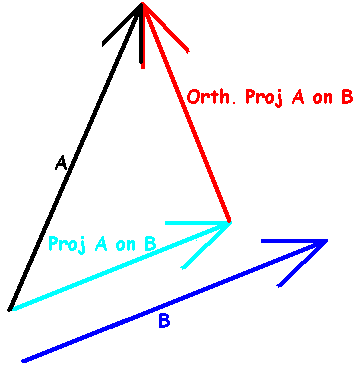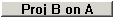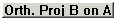# Projection

If you have two vectors, it can be useful to think of the first one as the sum of two vectors:

one vector in the same direction as the second vector, and

one vector perpendicular to the second vector.Here A is the first vector and B is the second. The light blue vector is in the same direction as B, and is called the Projection of A on B. The red vector is perpendicular to B; it can be called the Orthogonal Projection. To confuse things, often the projection itself is called the Orthogonal Projection.

CalGraph calculates both.

Enter the two vectors.

Clickto find the Projection of A onto B andto find the orthogonal projection.

You can find the Projection of B onto A, and its orthogonal projection usingand.

Projection of vectors only works if both vectors have the same dimension.